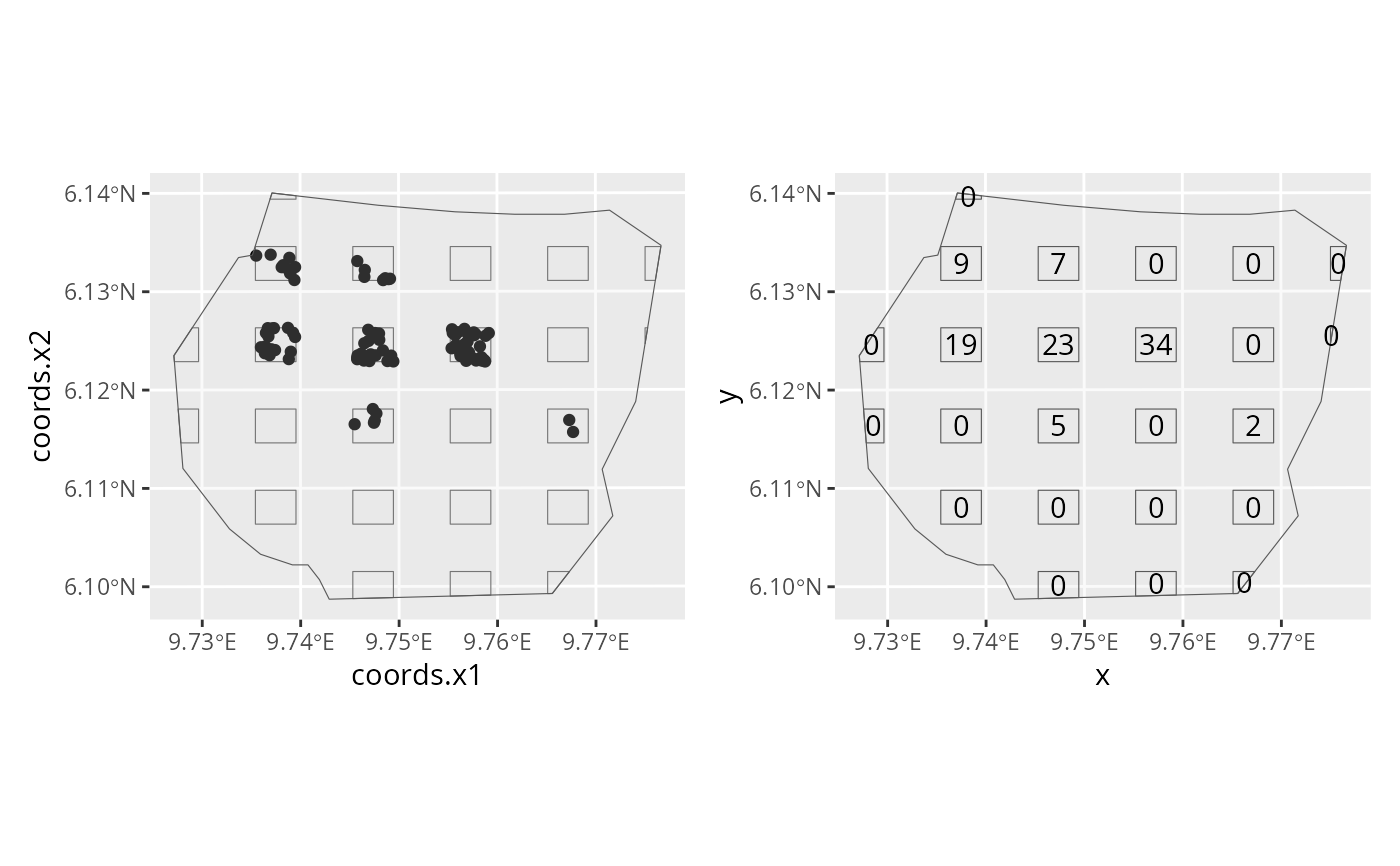Converts a plot sample with locations of each point within each plot, into a plot sample with only the count within each plot.

## Usage

point2count(plots, dets)

## Arguments

plots

A SpatialPolygonsDataFrame object containing the plots that were sampled.

dets

A SpatialPointsDataFrame object containing the locations of the points within the plots.

A SpatialPolygonsDataFrame with counts in each plot contained in slot @data$n. ## Examples # \donttest{ # Some features require the raster package if (require("raster", quietly = TRUE) && require("ggplot2", quietly = TRUE)) { data(gorillas, package = "inlabru") plotpts <- plotsample(gorillas$nests, gorillas$boundary, x.ppn = 0.4, y.ppn = 0.4, nx = 5, ny = 5 ) p1 <- ggplot() + gg(plotpts$plots) +
gg(plotpts$dets) + gg(gorillas$boundary)
countdata <- point2count(plotpts$plots, plotpts$dets)
x <- coordinates(countdata)[, 1]
y <- coordinates(countdata)[, 2]
count <- countdata@data$n p2 <- ggplot() + gg(gorillas$boundary) +
gg(plotpts\$plots) +
geom_text(aes(label = count, x = x, y = y))
multiplot(p1, p2, cols = 2)
}
#> Regions defined for each Polygons
#> Regions defined for each Polygons
#> Regions defined for each Polygons
#> Regions defined for each Polygons# }